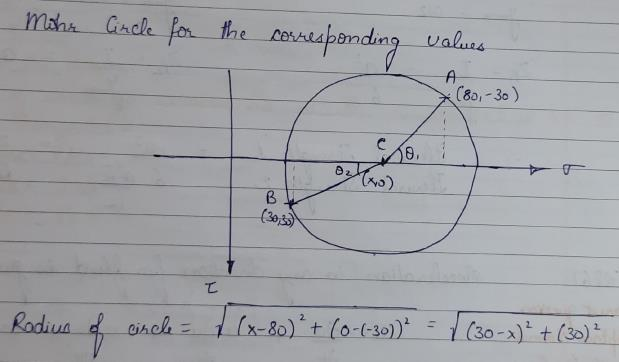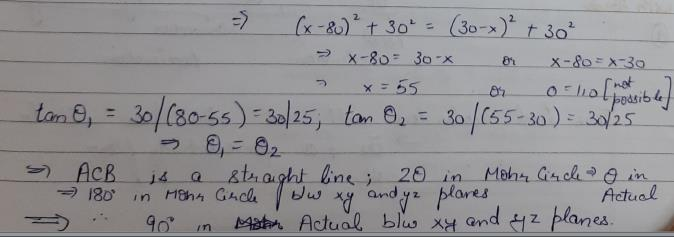Courses

# Test: Principal Stress & Strain - 2

## 10 Questions MCQ Test Solid Mechanics | Test: Principal Stress & Strain - 2

Description
This mock test of Test: Principal Stress & Strain - 2 for Civil Engineering (CE) helps you for every Civil Engineering (CE) entrance exam. This contains 10 Multiple Choice Questions for Civil Engineering (CE) Test: Principal Stress & Strain - 2 (mcq) to study with solutions a complete question bank. The solved questions answers in this Test: Principal Stress & Strain - 2 quiz give you a good mix of easy questions and tough questions. Civil Engineering (CE) students definitely take this Test: Principal Stress & Strain - 2 exercise for a better result in the exam. You can find other Test: Principal Stress & Strain - 2 extra questions, long questions & short questions for Civil Engineering (CE) on EduRev as well by searching above.
QUESTION: 1

### If the principal stresses at point in a strained body are p1 and p2 ( p1 > p2), then th e resu lta n t stress on a plane carrying the maximum shear stress is equal to

Solution: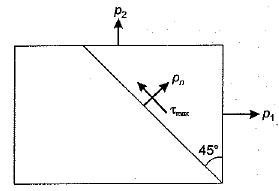Plane of maximum shear is at 45°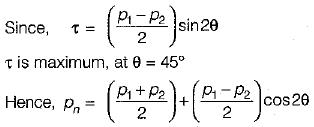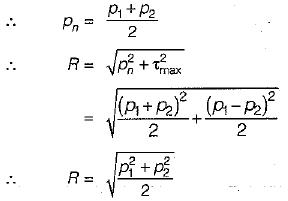QUESTION: 2

### If ∈1 and ∈2 ( ∈1 > ∈2) are the m axim um and minimum strains in the neighbourhood of a point in a stressed material of Young’s modulus E and Poisson’s ratio p, then the maximum principal stress will be given by

Solution: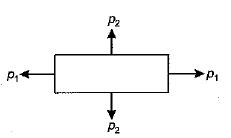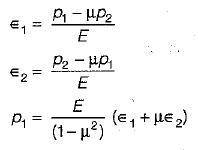QUESTION: 3

### On the element shown below in the figure, the stress in MPa are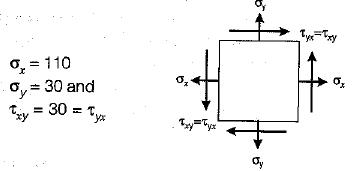The radius of Mohr’s circle V and principal stresses σ1, and σ2 are in (MPa) respectively

Solution: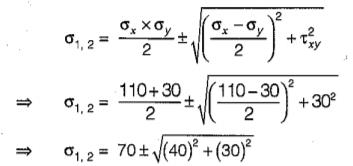⇒ σ1,2 = 70 ± 50
∴ σ1 = 120 MPa
and σ2 = 20 MPa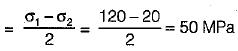QUESTION: 4

The radius of Mohr’s circle gives the value of

Solution:
QUESTION: 5

A body is subjected to two normal strains of magnitude ∈x = 0.003 and ∈y = 0.002. The shearing strain on a plane inclined at 30° with ∈x is

Solution:

Shearing strain
= (∈x - ∈y) sin2θ
= (0.003 - 0.002)sin60°
= √3/2 x 10-3

QUESTION: 6

Which one of the following Mohr’s circles represents the state of pure shear?

Solution:
QUESTION: 7

A bar is subjected to a uniaxial tensile stress ‘σ’. The tangential stress on a plane inclined at θ to the bar would be

Solution:

Normal sress = σ cos2 θ
Tangential Stress = σ sin θ cos θ

= σ sin 2θ/2

QUESTION: 8

Which of the following formulae is used to calculate tangential stress, when a member is subjected to stress in mutually perpendicular axis and accompanied by shear stress?

Solution:
QUESTION: 9

Normal stresses of equal magnitude a, but of opposite signs, act at a point of a strained material in perpendicular direction. What is the magnitude of the stress on a plane inclined at 45° to the applied stresses?

Solution: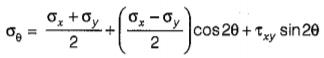Here, σx = σy = -σ and θ = 45°
∴ σθ = 0

QUESTION: 10

Two planes xyand yz are passing through a point in a strained material. The normal and shear stresses on xy plane are +80 MPa, -30 MPa respectively and normal and shear stresses on plane yz are +30 MPa and +30 MPa respectively. What is the angle between the planes?

Solution: